How to compute the impulse response of an eddy current problem?

Today, we will speak about eddy current problems. By the Wikipedia definition, eddy currents are loops of currents induced within conducting material by a changing magnetic field. Admittedly, there is some controversy within the electrical engineering community about whether or not this definition is really correct. But, for our purposes today, the term induced current will have to suffice.

In electrical machines, several parts may have – wanted or unwanted – currents induced within them. These include, for instance:

• Stator windings and rotor bars (or damper bars of a synchronous machine)
• Shaft of an induction machine
• Solid permanent magnets

### Geometric Nightmare

Of these examples, the first one – stator windings – can be especially tricky to analyze. This is due to simple arithmetics: there are so many of them. Indeed, all the other examples listed are if not solid volumes of conducting material, then at least large blocks. By contrast, the stator very often has at least ten or more conductors in a single slot. Often more.

Many, many, many more. See below for an example (that you’ve hopefully already seen here).

That’s what a stator winding may look like in real life. Hundreds of small wires bundled together.

And to model that kind of a winding with finite element analysis, each of those strands has to be meshed, i.e. represented by at least a dozen triangles each. The end result is often similar to what you can see below.

And since each node in the mesh represents an additional unknown to be solved, the computational model will get very heavy, very soon.

### Some Signal Processing for ya

However, there are some loopholes we might be able to exploit. (We are – I’m just trying to keep you folks in suspense for a few seconds longer.) Let’s focus our attention on a single slot of the machine for now. We can easily generalize the results for the entire problem later on, if needed.

The slot is what you can call a linear time-invariant system – or LTI. An LTI system is exactly what the name suggests. It is linear, since there is nothing to saturate in a slot consisting of copper, insulation, and air. And it is time-invariant, meaning it will behave the same way whether you measure it now or tomorrow.

And as any signal processing enthusiast can tell you, LTI systems are fully characterized by their impulse response function. Simply put, you whack the slot with an infinite force for an infinitely short time and measure how it reacts, and you know everything there is to know about said slot.

Alright alright, that doesn’t sound simple at all. But believe me, that is a useful piece of information. For instance, if we know the impulse response, we can use it to compute the eddy current losses for any arbitrary current waveform we can think of. This can be done by a convolution, which a very light operation computationally speaking, compared to solving an entire finite element problem.

Now, we only need to be able to determine this function.

### Infinite Responses

Before we proceed any further, some mathematical basics are necessary. To even begin computing the impulse response function, we will first have to definite what is responding to which.

Now, our example slot is governed by an equation that looks something like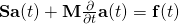,

where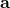is the unknown vector potential to be solved. Additionally, the boundary condition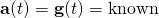has to be satisfied on the slot boundary.

To compute the impulse response function, this is what we need to do. We feed an impulse function – something that is infinitely high for an infinitely short time –  to e.g. the boundary value function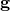, and see how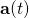responds to that.

#### Implementation Issues

This is where things get a bit difficult. The governing equation for the slot rarely has a nice closed-form solution, so we’ll have to use some numerical time-stepping method. And numerical methods do not handle infinite values very well.

Which is an optimistic way of saying “not at all”.

Well, we could utilize the other definition of the impulse response. In other words, we could compute the response of the system to a ramp function – something that grows linearly from zero – and then take the second time-derivative of that.

Which would lead to other numerical issues. Taking a second numerical derivative of anything will pretty much always lead to some nasty inaccuracies.

So, this approach doesn’t seem much better. It’s not exactly a dead end, but it’s not much better either.

#### Hybrids. Like X-files an’ stuff

However…what if we could try a hybrid approach? What if we could use the ramp-function thingie somehow to compute the initial conditions for the impulse function thingie?

In other words, if we could somehow determine what the system looks like immediately after the impulse function has passed, we could continue from that using a suitable time-stepping scheme. That would rid us of having to deal with the infinite input and double derivatives both.

Let’s do exactly this.

#### Maths. Enjoy.

We use the backward-Euler method to solveafter a single time step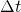. Denoting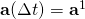, we can write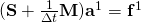.

To handle the boundary conditions, we splitinto its inner and boundary components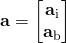.

By applying a similar division to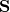and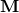, we get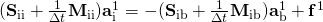where of course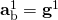.

Next, we set the time-dependency ofto the ramp function, or simply. Thus, the right-hand side can be denoted with one constant and one time-dependent term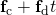.

Then, let’s write the inverse of the left-hand side matrix with a matrix Neumann series, i.e. with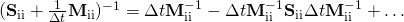By multiplying the right-hand side with this series expansion lets us write the solution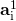as a power series of.

Note that this series expansion is valid for all time-step lengths. Thus, we can use it to compute the desired initial condition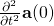,

simply by taking the second derivative of the series expansion and lettingapproach zero.

Indeed, we see that we only need one term of the series expansion for this purpose – the one corresponding to the second power of. That one will be constant after two derivatives, and hence won’t go to zero whendoes.

#### More Math. You’re Welcome.

Like the TV infomercial said – this is not all!

This is not mechanical engineering what we’re dealing with, so the matrixis typically not invertible. This is due to the fact that only a part of the slot is electrically conductive, but I digress. Furthermore, we often have some kind of a circuit connections in our problem too.

Combining all these factors leads us to a system of type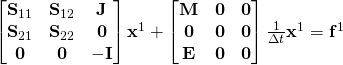where the unknown vector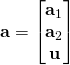contains the conductive and nonconductive components ofand the voltages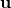respectively. Additionally, both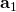andshould be divided into their inner and boundary terms as before, but I’ve omitted that step for clarity.

Next, we write the problem as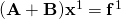,

where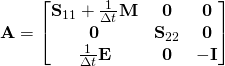and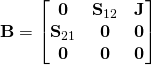The Neumann series approach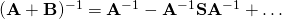can then be utilized for this problem in exactly the same fashion as before. It’s an exercise in patience and pain, but it can be done, mind you.

#### Even More Math. Forgive me.

Please note that the solution you get with this approach is never the true impulse response. This is because a true impulse response function would have infinitely thin surface current densities on the borders of the conducting regions. Obviously, these current sheets cannot be represented by a mesh of finite density.

Hence, some error is inevitable in the physical space. But, in the time-domain – how the system behaves as a function of time – should be exact. Meaning, the behaviour computed with the impulse response function and the convolution should be the same as you’d get by a brute-force numerical analysis.

Moreover, note that in the latter case – with voltages included – there might be constant terms in the solution series. Even terms proportional to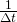if you happen to be interested in boundary fluxes (don’t ask…). These terms translate to impulse functions and unit doublet functions in the impulse response, respectively.

### Results

After this exhausting derivation, we can finally compute the desired impulse response. We obtain the initial conditions with the series approach is just described, from which we can continue with typical time-stepping methods.

Below, you can see an example of the initial conditions. The block on the right is conductive, whereas the left side isn’t. An impulse is fed to the left-side boundary, inducing a current density on the boundary of the conductive block.

This current density is denoted with the nice colors in the figure. Per Lenz’s law, it prevents the flux from penetrating very deep into the conducting material.

Edit: For a nice explanation of Lenz’s law, check out this site here.

Of course, this is a highly simplified example here. But, I promise to show you something more interesting later.

Until then!

-Antti

Check out EMDtool - Electric Motor Design toolbox for Matlab.

Need help with electric motor design or design software? Let's get in touch - satisfaction guaranteed!Faster Analysis of Eddy-Current Problems: Impulse Response Method